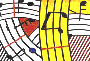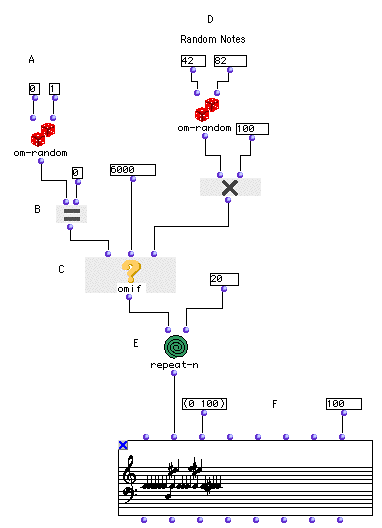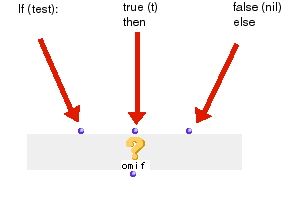Tutorial 14 - Random construction of a sequence III

Introduction to omif

Topics

Introduction to conditional functions and predicates.

Functions used

om-if and om=Description

This patch uses omif module for conditional control with the equality test om=.

Patch structure

A: We will use here om-random as a 'heads and tail' coin draw generating zeros and 1.

B: om= will test the output of om-random with its second argument 0. If om-random outputs a zero the test will be true and will return the Boolean lisp value t. If the output of om-random is 1 om= will return nil for false.

C: When omif receives a ' t' value in its first input, the second input will be evaluated. Else (in the case of 'nil' value) omif will evaluate its third input.In our example, we have choosen to input one value (6000 = C3) as a fixed note whenever om-random (A) will output a zero. If 1 is outputed, omif will evaluate the D patch which is a random note generating patch which is identical to the one in tutorial 12.

E: repeat-n will evaluate n-times the whole patch (in this example 20 times) and output its result to the CHORD-SEQ (F).